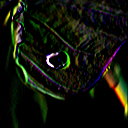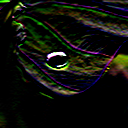### Linear Spatial Image Filtering

The Generic Scene Node Composer provides the following two nodes to perform linear spatial filtering with custom filter kernels:
• ImageProcessing.Compute.Filter.Filter
• ImageProcessing.Compute.Filter.Separable

### ImageProcessing.Compute.Filter.Filter

Linear spatial filtering is a versatile method for image filtering and can achieve many effects, such as blurring, sharpening, embossing, outlining, etc. Mathematically, linear spatial filter can be described by a 2D convolution operation.

This node performs filtering by computing a 2D convolution of an input image $\mathrm{a}[x,y]$ and an image kernel $\mathrm{k}[x,y]$ resulting in an output image $\mathrm{f}[x,y]$:
$\mathrm{f}[x,y] = \mathrm{a}[x,y] \ast \mathrm{k}[x,y] = \sum\limits_{j = -\infty}^{\infty} \sum\limits_{k = -\infty}^{\infty} \mathrm{k}[j, k] \, \,\mathrm{a}[x-j,y-k]$
Dependent on the selected image kernel $\mathrm{k}[x,y]$, which can be set via the node's input slot labeled kernel, different filtering results can be achieved.

The output image $\mathrm{f}[x,y]$ at slot output has the same size as the input image $\mathrm{a}[x,y]$ at slot input. To evaluate the equation for the 2D convolution, all kernel values $\mathrm{k}[x,y]$ outside the provided image size are assumed to be zero. Furthermore, if the convolution requires input image pixel values outside of the provided image size, the x-/y-positions are clamped to the spatially closest one on the border of the image (clamp to border).

#### Example

In order to get a better understanding of the 2D convolution, the figure below visualize the 2D convolution for a simple example, in which an input image $\mathrm{a}[x,y]$ of size 4 x 3 pixel is convolved with a kernel $\mathrm{k}[x,y]$ of size 3 x 3 pixel. If the kernel is assumed to be zero outside the provided spatial range and if the kernel's origin is located at its center pixel, the 2D convolution for this example simplifies to:

$\mathrm{f}[x,y] = \mathrm{a}[x,y] \ast \mathrm{k}[x,y] = \sum\limits_{j = -1}^{1} \sum\limits_{k = -1}^{1} \mathrm{k}[j, k] \, \,\mathrm{a}[x-j,y-k]$

Thus, for a 3 x 3 kernel, for each output pixel $(x,y)$ we need to compute the weighted sum of the input image value at pixel position $(x,y)$ and the values of its 8 neighboring pixels (9 additions in total), whereby the multiplicative weights are given by the provided kernel (mirrored in x- and y-direction).Kernel $k[x,y]$
Mirrored kernel
$x$
$y$
$-1$
$0$
$1$
$-1$
$0$
$1$
$x$
$y$
$1$
$0$
$-1$
$1$
$0$
$-1$
Input image $a[x,y]$
Padded input image (clamp to border)
$x$
$y$
$0$
$1$
$2$
$0$
$1$
$2$
$3$
Ouput image $f[x,y]$
Here are a few examples for different 3 x 3 kernels and their results:Identity (no filtering) $\begin{bmatrix}0 & 0 & 0\\ 0 & 1 & 0\\ 0 & 0 & 0 \end{bmatrix}$Binomial blur filter $\frac{1}{16}\begin{bmatrix}1 & 2 & 1\\ 2 & 4 & 2\\ 1 & 2& 1 \end{bmatrix}$Sharpening $\begin{bmatrix}-1 & -1 & -1\\ -1 & 9 & -1\\ -1 & -1& -1 \end{bmatrix}$Gradient X $\begin{bmatrix}1 & 0 & -1\\ 2 & 0 & -2\\ 1 & 0& -1 \end{bmatrix}$Gradient Y $\begin{bmatrix}1 & 2 & 1\\ 0 & 0 & 0\\ -1 & -2& -1 \end{bmatrix}$Emboss $\begin{bmatrix}-2 & -1 & 0\\ -1 & 1 & 1\\ 0 & 1& 2 \end{bmatrix}$

The graph Filter3x3 demonstrates this examples.

### ImageProcessing.Compute.Filter.Separable

This nodes performs linear spatial filtering with custom filter kernels. In contrast to the filter node described above, this node does not execute a convolution with a 2D kernel. Instead two 1D kernels in x- and y-direction are applied. If a 2D filter $\mathrm{k}[x,y]$ is realizable by two 1D filters, it is called separable. Mathematically, this can be described as:

$\mathrm{f}[x,y] = \mathrm{a}[x,y] \ast \mathrm{k}[x,y] = \mathrm{a}[x,y] \ast \mathrm{k}_x[x] \ast \mathrm{k}_y[y]$
where $\mathrm{k}_x[x]$ is the kernel in x-direction given via the node's input slot kernelX and $\mathrm{k}_y[y]$ is the kernel in y-direction at input slot kernelY.
As an example, the 3 x 3 gradient filter from the example above can be realized by two 1D kernels:
$\mathrm{f}[x,y] = \mathrm{a}[x,y] \ast \begin{bmatrix}1 & 0 & -1\\ 2 & 0 & -2\\ 1 & 0& -1 \end{bmatrix} = \mathrm{a}[x,y] \ast \begin{bmatrix}1 & 0 & -1\end{bmatrix} \ast \begin{bmatrix}1\\ 2\\ 1\\\end{bmatrix}$
The 2D kernel in this example would need to sum up 9 elements, whereas the two 1D kernels in total only 6. Therefore, a separable filter implemetation is computationally more efficient (and the difference becomes even more significant if larger kernels are employed). The only disadvantage is that the separable implementation requires more memory because an intermediate output image needs to be temporally stored.
The kernel in x-direction at slot kernelX is specified by an image with a height of 1 pixel. Mathematically it would be consistent if the kernel in y-direction would be specified by an image with a width of 1 pixel. However, this would make it more complicated in situations where the kernel in x- and y-direction should be the same, as it is the case, for example, for a binomial blur filter:
$\mathrm{f}[x,y] = \mathrm{a}[x,y] \ast \frac{1}{16}\begin{bmatrix}1 & 2 & 1\\ 2 & 4 & 1\\ 1 & 2& 1 \end{bmatrix} = \mathrm{a}[x,y] \ast \frac{1}{4}\begin{bmatrix}1 & 2 & 1\end{bmatrix} \ast \frac{1}{4}\begin{bmatrix}1\\ 2\\ 1\\\end{bmatrix}$
Therefore, the kernel in y-direction at slot kernelY is also specified by an image with a height of 1 pixel and the required transformation in y-direction is performed internally.

The example Separable demonstrates how to use this node.